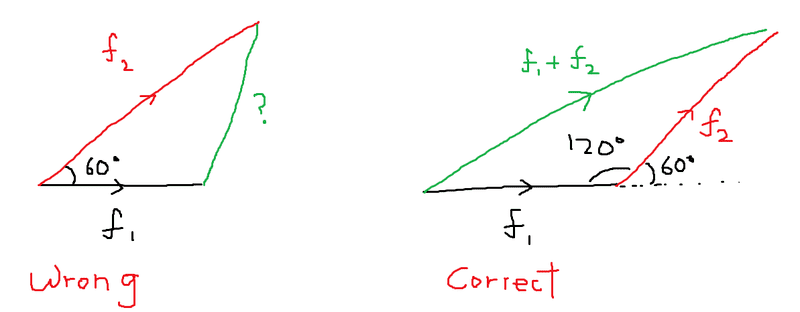# Find resultant vector and equilibrant

• B
• rrosa522

#### rrosa522

A force, f1, of a magnitude 6 N acts on particle P. A second force, f2, of magnitude 8 N acts at 60 degrees to f1. Determine the resultant and equilibrant of f1 and f2.

I use cosine law and found the resultant is 7.2 but the answer is 12.7
I used angle 60 and my solution manual says I had to use 120, but I don't understand why that it

You did your vector addition wrongly. Try drawing out the two vectors f1 and f2 as well as their resultant vector.

You did your vector addition wrongly. Try drawing out the two vectors f1 and f2 as well as their resultant vector.
i did but i am not getting the right answer

i did but i am not getting the right answer
I'm quite sure you drew both vectors as having the same origin (i.e. tails originating from the same point) and then trying to find the line that completes that triangle. This is not correct. The tail of one vector has to start from the arrowhead of the other.

(I'm kinda lazy to attempt to sketch figures - so take a look over at http://hyperphysics.phy-astr.gsu.edu/hbase/vect.html)

I'm quite sure you drew both vectors as having the same origin (i.e. tails originating from the same point) and then trying to find the line that completes that triangle. This is not correct. The tail of one vector has to start from the arrowhead of the other.

(I'm kinda lazy to attempt to sketch figures - so take a look over at http://hyperphysics.phy-astr.gsu.edu/hbase/vect.html)
yeah that is what I did, I just don't get why its cos120 and not cos60. the question says A second force, f2, of magnitude 8 N acts at 60 degrees to f1, doesn't that mean f1 and f2 form an angle of 60 degrees.What you probably drew is the incorrect diagram on the left.